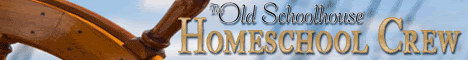### Math Galaxy

Math Galaxy is a software program for grades K-12. It is designed to connect "the abstract concepts of math to concrete experiences with graphical illustrations at each step to help you remember." Detailed explanations are given and practice opportunities are unlimited with this computer math program.

Each of the following programs are available as a CD-ROM:
• Math Galaxy Whole Numbers Fun
• Math Galaxy Fractions Fun
• Math Galaxy Decimals, Proportions, % Fun
• Math Galaxy Word Problems Fun
• Math Galaxy Pre-Algebra Fun
• Algebra Fundamentals
• Whole Numbers Worksheet Generator
• Fractions Worksheet Generator
• Decimals, Proportions, % Worksheet Generator
• Algebra Worksheet Generator

You can check out the website for a detailed description of each one, or Tour Math Galaxy to see more.

Price: \$29.95 for each CD-ROM.

Two eBook selections are also available for \$14.95 each:
• Math Galaxy Whole Number Riddles eBook
• Math Galaxy Fraction Riddles eBook